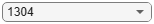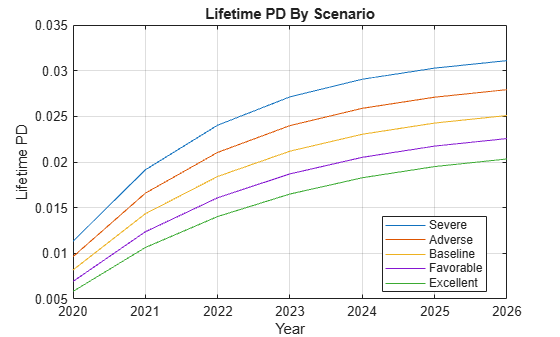# Expected Credit Loss Computation

This example shows how to perform expected credit loss (ECL) computations with `portfolioECL` using simulated loan data, macro scenario data, and an existing lifetime probability of default (PD) model.

Load loan data ready for prediction, macro scenario data, and corresponding scenario probabilities.

```load DataPredictLifetime.mat disp(LoanData)```
``` ID ScoreGroup YOB Year ____ _____________ ___ ____ 1304 "Medium Risk" 4 2020 1304 "Medium Risk" 5 2021 1304 "Medium Risk" 6 2022 1304 "Medium Risk" 7 2023 1304 "Medium Risk" 8 2024 1304 "Medium Risk" 9 2025 1304 "Medium Risk" 10 2026 2067 "Low Risk" 7 2020 2067 "Low Risk" 8 2021 2067 "Low Risk" 9 2022 2067 "Low Risk" 10 2023 ```
`disp(head(MultipleScenarios,10))`
``` ScenarioID Year GDP Market __________ ____ ____ ______ "Severe" 2020 -0.9 -5.5 "Severe" 2021 -0.5 -6.5 "Severe" 2022 0.2 -1 "Severe" 2023 0.8 1.5 "Severe" 2024 1.4 4 "Severe" 2025 1.8 6.5 "Severe" 2026 1.8 6.5 "Severe" 2027 1.8 6.5 "Adverse" 2020 0.1 -0.5 "Adverse" 2021 0.2 -2.5 ```
`disp(ScenarioProbabilities)`
``` Probability ___________ Severe 0.1 Adverse 0.2 Baseline 0.3 Favorable 0.2 Excellent 0.2 ```
```load LifetimeChampionModel.mat disp(pdModel)```
``` Probit with properties: ModelID: "Champion" Description: "A sample model used as champion model for illustration purposes." UnderlyingModel: [1x1 classreg.regr.CompactGeneralizedLinearModel] IDVar: "ID" AgeVar: "YOB" LoanVars: "ScoreGroup" MacroVars: ["GDP" "Market"] ResponseVar: "Default" WeightsVar: "" ```

For ECL computations, only the marginal PDs are required. However, first you can visualize the lifetime PDs.

```CompanyIDChoice ="1304"; CompanyID = str2double(CompanyIDChoice); IndCompany = LoanData.ID == CompanyID; Years = LoanData.Year(IndCompany); NumYears = length(Years); ScenarioID = unique(MultipleScenarios.ScenarioID,'stable'); NumScenarios = length(ScenarioID); LifetimePD = zeros(NumYears,NumScenarios); for ii=1:NumScenarios IndScenario = MultipleScenarios.ScenarioID==ScenarioID(ii); data = join(LoanData(IndCompany,:),MultipleScenarios(IndScenario,:)); LifetimePD(:,ii) = predictLifetime(pdModel,data); end plot(Years,LifetimePD) xticks(Years) grid on xlabel('Year') ylabel('Lifetime PD') title('Lifetime PD By Scenario') legend(ScenarioID,'Location','best')```### Compute ECL

The computation of ECL requires a marginal PD values, LGD values, and EAD values, effective interest rate, plus the scenarios and scenario probabilities.

Compute the lifetime ECL using the `portfolioECL` function. The inputs to this function are tables, where the first column is an ID variable that indicates which rows correspond to which loan. Because the projections cover multiple periods for each loan, and the remaining life of different loans may be different, the ID variable is an important input. For each ID, the credit projections must be provided, period-by-period, until the end of the life of each loan. Typically, the marginal PD has a multi-period and multi-scenario size. This example assumes constant LGD and EAD values. This means that the same LGD and EAD is used for all periods, and the LGD and EAD values are not sensitive to the scenarios. Hence, the marginal PD input has multiple rows and columns per ID, whereas the LGD and EAD inputs have one scalar value per ID. To offer flexibility for different input dimensions for marginal PD, LGD, and EAD inputs, these inputs are separated into three separate tables in the syntax of `portfolioECL`.

```ScenarioID = unique(MultipleScenarios.ScenarioID,'stable'); NumScenarios = length(ScenarioID);```

Predict marginal PD for each scenario. The `predictLifetime` function is called for the entire portfolio at once, and the marginal PDs for each scenario are stored as columns.

```MarginalPD = zeros(height(LoanData),NumScenarios); for ii=1:NumScenarios IndScenario = MultipleScenarios.ScenarioID==ScenarioID(ii); data = join(LoanData,MultipleScenarios(IndScenario,:)); MarginalPD(:,ii) = predictLifetime(pdModel,data,'ProbabilityType','marginal'); end```

Convert to the required table input format, with the ID column.

```MarginalPDTable = array2table(MarginalPD); MarginalPDTable.Properties.VariableNames = ScenarioID; MarginalPDTable = addvars(MarginalPDTable,LoanData.ID,'Before',1,'NewVariableNames','ID'); disp(MarginalPDTable)```
``` ID Severe Adverse Baseline Favorable Excellent ____ __________ __________ __________ __________ __________ 1304 0.011316 0.0096361 0.0081783 0.006918 0.0058324 1304 0.0078277 0.0069482 0.0061554 0.0054425 0.0048028 1304 0.0048869 0.0044693 0.0040823 0.0037243 0.0033938 1304 0.0031017 0.0029321 0.0027698 0.0026147 0.0024668 1304 0.0019309 0.0018923 0.0018538 0.0018153 0.001777 1304 0.0012157 0.0012197 0.0012233 0.0012264 0.0012293 1304 0.00082053 0.00082322 0.00082562 0.00082775 0.00082964 2067 0.0022199 0.001832 0.0015067 0.001235 0.0010088 2067 0.0014464 0.0012534 0.0010841 0.00093599 0.00080662 2067 0.0008343 0.00074897 0.00067168 0.00060175 0.00053857 2067 0.00049107 0.00045839 0.00042769 0.00039887 0.00037183 ```

The LGD and EAD table inputs are small tables with one row per ID.

```UniqueIDs = unique(LoanData.ID,'stable'); NumIDs = length(UniqueIDs); LGD = 0.55; LGDTable = table(UniqueIDs, repmat(LGD,NumIDs,1),'VariableNames',{'ID','LGD'}); disp(LGDTable)```
``` ID LGD ____ ____ 1304 0.55 2067 0.55 ```
```EAD = 100000; EADTable = table(UniqueIDs, repmat(EAD,NumIDs,1),'VariableNames',{'ID','EAD'}); disp(EADTable)```
``` ID EAD ____ _____ 1304 1e+05 2067 1e+05 ```

For simplicity, assume the same effective interest rate for both loans.

`EffRate = 0.045;`

Call the `portfolioECL` function. The first output is the total ECL, or provisions, for the portfolio.

```[totalECL, ECLByID, ECLByPeriod] = portfolioECL(MarginalPDTable, LGDTable, EADTable, 'InterestRate', EffRate,... 'ScenarioNames',ScenarioID, 'ScenarioProbabilities',ScenarioProbabilities.Probability, 'IDVar','ID','Periodicity','annual'); fprintf('Total portfolio lifetime ECL is: %.2f\n',totalECL)```
```Total portfolio lifetime ECL is: 1401.00 ```

The second output, `ECLByID`, shows the ECL for each ID. The third output, `ECLByPeriod`, shows the ECL for each period, and each scenario. Use the dropdown to select an ID and display the corresponding ECL information.

```CompanyIDChoice ="1304"; CompanyID = str2double(CompanyIDChoice); disp(ECLByID(ECLByID.ID==CompanyID,:))```
``` ID ECL ____ ______ 1304 1217.3 ```
`disp(ECLByPeriod(ECLByPeriod.ID==CompanyID,:))`
``` ID TimePeriod Severe Adverse Baseline Favorable Excellent ____ __________ ______ _______ ________ _________ _________ 1304 1 595.58 507.16 430.44 364.11 306.97 1304 2 394.24 349.95 310.02 274.11 241.9 1304 3 235.53 215.4 196.75 179.5 163.57 1304 4 143.05 135.23 127.75 120.59 113.77 1304 5 85.219 83.517 81.816 80.118 78.429 1304 6 51.346 51.514 51.665 51.798 51.917 1304 7 33.162 33.271 33.368 33.454 33.531 ```

For more information, see the Incorporate Macroeconomic Scenario Projections in Loan Portfolio ECL Calculations example that shows a detailed workflow for ECL calculations, including the determination of macro scenarios, the use of lifetime PD, LGD and EAD models, and a visualization of credit projections and provisions for each ID to drill down to a loan level.